Cheng, L., Wang, W., Yang, Z., and Dai, J. (2020). "Establishing a dynamic elastic modulus prediction model of larch based on nondestructive testing data," BioRes. 15(3), 4835-4850.

#### Abstract

To accurately evaluate the dynamic elastic modulus (Ed) of wood in ancient timberwork buildings, the new materials of larch were used as the research object, and the stress wave nondestructive testing method was used to determine it. Based on nondestructive testing data, this paper proposed a method for predicting the Ed of larch using the principle of information diffusion. It selected the distance (D) from the bark to the pith in the cross-section of the wood and the height (H) from the base to the top in the radial section of the wood. The fuzzy diffusion relationships between the two evaluation indexes and the Ed were established using the information diffusion principle and the first- and second-order fuzzy approximate inferences in the fuzzy information optimization process. The calculation results showed that the dynamic elastic modulus model constructed by the information diffusion method can better predict the Ed of larch. The coefficient of determination between the measured value and the predicted value of the Ed was 0.861, they were in good agreement. The weights of the two influencing factors were 0.7 and 0.3, respectively, the average relative error of the fitted sample data was the minimum, which was 8.55%. This prediction model provided a strong basis for field inspection.

Establishing a Dynamic Elastic Modulus Prediction Model of Larch Based on Nondestructive Testing Data

Liting Cheng,a Wei Wang b,c,d,* Zhiguo Yang,e and Jian Dai,b,c,d,*

To accurately evaluate the dynamic elastic modulus (Ed) of wood in ancient timberwork buildings, the new materials of larch were used as the research object, and the stress wave nondestructive testing method was used to determine it. Based on nondestructive testing data, this paper proposed a method for predicting the Ed of larch using the principle of information diffusion. It selected the distance (D) from the bark to the pith in the cross-section of the wood and the height (H) from the base to the top in the radial section of the wood. The fuzzy diffusion relationships between the two evaluation indexes and the Ed were established using the information diffusion principle and the first- and second-order fuzzy approximate inferences in the fuzzy information optimization process. The calculation results showed that the dynamic elastic modulus model constructed by the information diffusion method can better predict the Ed of larch. The coefficient of determination between the measured value and the predicted value of the Ed was 0.861, they were in good agreement. The weights of the two influencing factors were 0.7 and 0.3, respectively, the average relative error of the fitted sample data was the minimum, which was 8.55%. This prediction model provided a strong basis for field inspection.

Keywords: Stress wave; Distance base-top; Fuzzy matrix; Information diffusion

Contact information: a: College of Architecture and Civil Engineering, Beijing University of Technology, Beijing 100124, China; b: College of Architecture and Urban Planning, Beijing University of Technology, Beijing 100124, China; c: Beijing Engineering Technology Research Center for Historic Building Protection, Beijing University of Technology, Beijing 100124, China; d: Key Science Research Base of Safety Assessment and Disaster Mitigation for Traditional Timber Structure (Beijing University of Technology), State Administration for Cultural Heritage, Beijing 100124,China; e: College of Petroleum Engineering, China University of Petroleum (Beijing), Beijing 102249, China;

*Corresponding author: chenglting@126.com; ieeww@bjut.edu.cn

INTRODUCTION

Wood is an important renewable natural resource and is widely used in construction, furniture, and bridges (Hou 2019). Because wood occupies an important position in ancient timberwork buildings, it has become one of the important goals of wood research to effectively, quickly, and accurately test the timber property of the wooden structure on site without damaging the material itself and the original structure of the ancient wooden structure members (Li 2015). Among the wood property indexes, elastic modulus is an important wood property index that can reflect the wood’s ability to change under load (Tian et al. 2017; Cavalheiro et al. 2018). At the same time, it is an important basis for nondestructive testing of wood and automatic classification of wood strength (Wang and Zhang 2006). Therefore, accurately predicting the elastic modulus of wood has an important practical significance. The traditional elastic modulus detection method is through mechanical experiments, which are destructive and not suitable for ancient buildings. In recent years, nondestructive testing technologies have been widely used in the field of wood property testing (Biechele et al. 2011; Ming et al. 2013; Chang 2017). The nondestructive testing methods currently applied to the determination of the elastic modulus of wooden components of ancient buildings are: ultrasonic method (Moreno-Chan et al. 2011), stress wave technology (Guan et al. 2013; Menezzi et al. 2014), SilviScan (Xu et al. 2012; Dahlen et al. 2018), and micro-drilling resistance instrument technology (Zhu et al. 2011; Sun 2012; Sun et al. 2012). These techniques have been widely used in nondestructive testing of wood properties (Zhu 2012; Dackermann et al. 2016; Wang et al. 2016; Haseli et al. 2020). It is of great practical significance to predict the elastic modulus index of the whole wood by using a reasonable calculation model, based on nondestructive testing technology to detect theof wood.

Information diffusion (Huang 2005; Hao et al. 2010) is a fuzzy mathematical processing method for set-valued samples that considers the optimal use of sample fuzzy information to make up for insufficient information. It turns a sample point of distinct values into a fuzzy set (Wang et al. 2019). It is built and developed on the basis of information distribution methods, the basic idea is to directly transfer the original information to the fuzzy relationship in a certain way, thereby avoiding the calculation of the membership function, so as to retain the original information carried by the original data to the greatest extent possible (Huang and Wang 1995). Information diffusion can be divided into two ways: one is to assign single-value samples to different discrete points to achieve data fuzziness, and the other is to find the information matrix determined by the discrete points of the two universes to get a fuzzy relationship between the two (He et al. 2008). This article adopted the second way to build a prediction model.

It is key to accurately detect the properties of wooden members for correctly evaluating the bearing capacity and service life of ancient wooden structure members. This has been proved in many on-site nondestructive testing practices. Based on the principle of information diffusion and the original data obtained by nondestructive testing technology, this paper established the distance (D) between the larch wood from the bark to the pulp pith, the height (H) from the base to the top of the tree, and the dynamic elastic modulus forecasting model. During on-site operation, determining the actual location of the measurement point on the cross-section and longitudinal section firstly, then, checking the Ed, collecting and summarizing all the results, removing the error value points, and selecting the proper weight value, then the Ed of the component is obtained. This study hopes to provide a basis and reference value for the rapid and accurate detection of the dynamic elastic modulus of ancient wooden structure members.

EXPERIMENTAL

Materials

The experimental materials were selected from larch (Larix gmelinii) materials that were above the fiber saturation point (FSP). Materials were purchased from Qingdongling Timber Factory (Tangshan City, Hebei Province, China). The diameter of the base was 50 cm, the diameter of the top was 40 cm, and the height was 400 cm. According to the number of annual rings and the information provided by the factory, the wood was approximately 300 to 400 years old. The wood was cut from the base to the top every 50 cm and was divided into 8 sections. The sections were labeled A, B, C, D, E, F, G, H and the shaded and light sides were marked (Figs. 1a, b, c). According to the standard GB 1929 (2009) for selecting test specimens, each section was sawn into 2 cm × 2 cm × 45 cm specimens. A total of 559 flawless specimens were selected. The test specimens were divided into 10 groups according to the actual depth (Tables 1a, b). Among these, group 1 was the pith, and group 10 was the bark. The number of specimens in each group is shown in Table 2. Group A was the base, and group H was the top.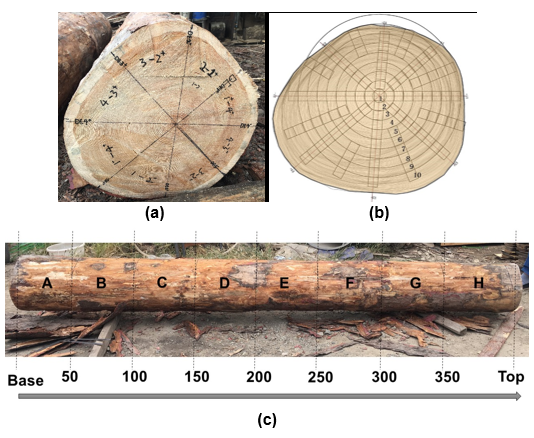Fig. 1. Schematic diagram of cross-section and radial sections: (a) schematic diagram of cross-section, (b) schematic diagram of cross-section interception, and (c) schematic diagram of radial section

Table 1(a). Groups Listed by Depth in Cross-section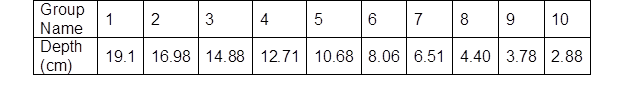Table 1(b). Groups Listed by Height in Radial SectionTable 2. Number of Specimens in Each Group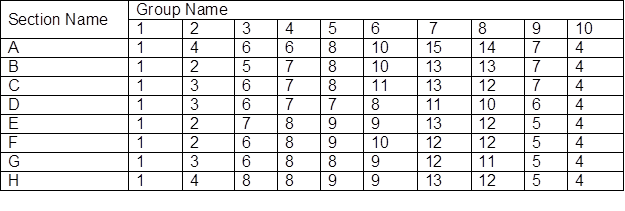Equipment

The apparatuses used were a Lichen Technology blast dryer box 101-3BS (Shanghai Lichen Electronic Technology Co., Ltd., Shanghai, China), Lichen Technology electronic precision balance JA1003 (Shanghai Lichen Electronic Technology Co., Ltd., Shanghai, China), Vernier calipers (Guilin Guanglu Measuring Instrument Co., Ltd., Guilin, China), and FAKOPP microsecond timber (FAKOPP Enterprise Bt., Ágfalva, Hungary)( Figs. 2a, b, c, d).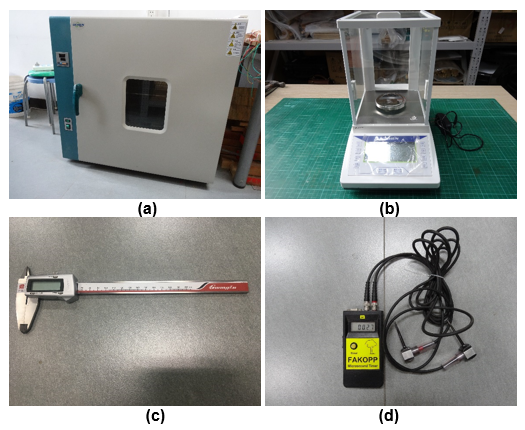Fig. 2. Experiment equipment– (a) dryer box; (b) electronic precision balance; (c) Vernier calipers; and (d) FAKOPP microsecond timber

The propagation time of stress wave in wood was measured with a stress wave tester FAKOPP (FAKOPP Enterprise Bt., Ágfalva, Hungary). The two probes of the instrument were inserted into both ends of the test specimens and the propagation time was measured along the longitudinal direction of the test specimens. The angle between the two probes and the length of the test specimens was not less than 45°, and the distance between the two measurement points was measured, as shown in Fig. 3. During the measurement, the reading of the propagation time of the first tap was invalid. Starting from the second time, the average value of the propagation time obtained by continuously measuring three times was used as the final test result. According to the application principle of the stress wave tester (Zhang and Wang 2014), the propagation velocity and dynamic modulus of elasticity of the wood (Ed) were calculated using Eqs. 1 and 2. According to the actual position of specimens and the division method of the group, the average in the group was taken as the value of the stress wave propagation velocity and the Ed, see Table 3,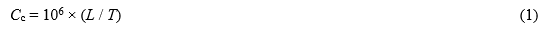where L is the distance between the two sensors of the stress wave tester (m), T is the time recorded between the two sensors of the stress wave tester (μs), and Cc is the stress wave propagation velocity in wood (m/s). Equation 2 is as follows,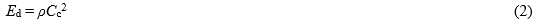where Ed is the dynamic modulus of elasticity of wood (MPa), ρ was the density of wood (g/cm3).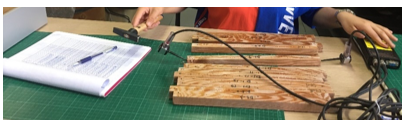Fig. 3. Measuring the stress wave propagation time

Table 3. Dynamic Elastic Modulus of Wood at Each Location Measured by Nondestructive Testing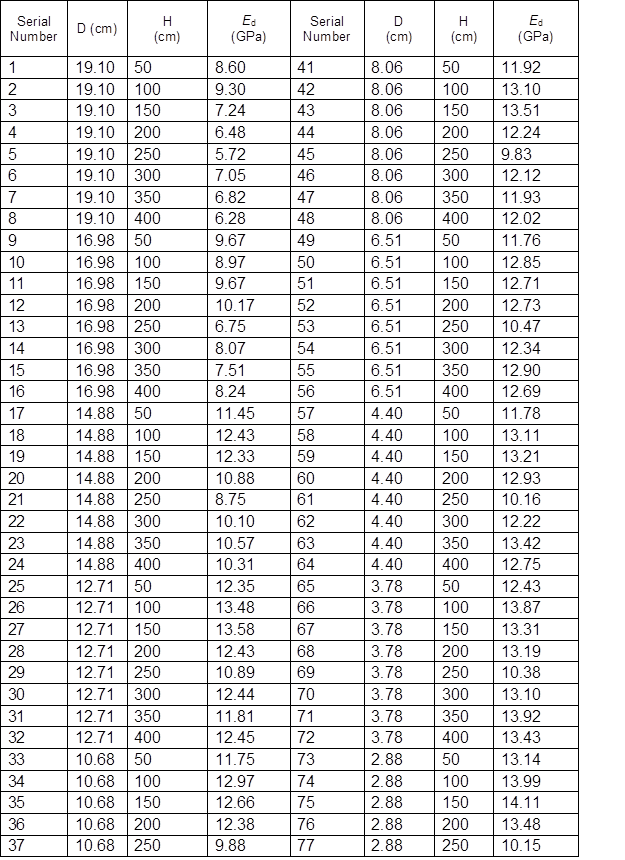Next, to determine the density and the moisture content (MC). According to Fig. 4 to cut off the specimens, the size was 2 cm × 2 cm × 2 cm. It was measured with a Vernier caliper (Guilin Guanglu Measuring Instrument Co., Ltd., Guilin, China), and the mass of the test specimens was recorded with a balance. The density calculation formula, ρ = M / V, was then used to calculate the density of all the test specimens. Where, M was the mass (g), V was the volume (cm3). According to the actual position of specimens and the division method of the group, the average in the group was taken as the value of the density.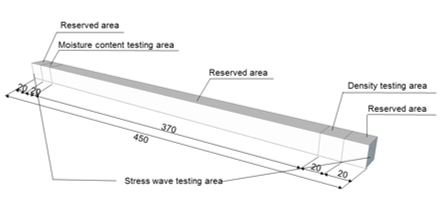Fig. 4. Experimental specimen partition (units are in mm)

The MC of the test specimens was then determined according to the GB 1931 (1991) standard (Fig. 5). After drying in a drying box, the mass of the wooden block was measured, and the MC was calculated. The measurement results showed that the MC was 4% ~7%.